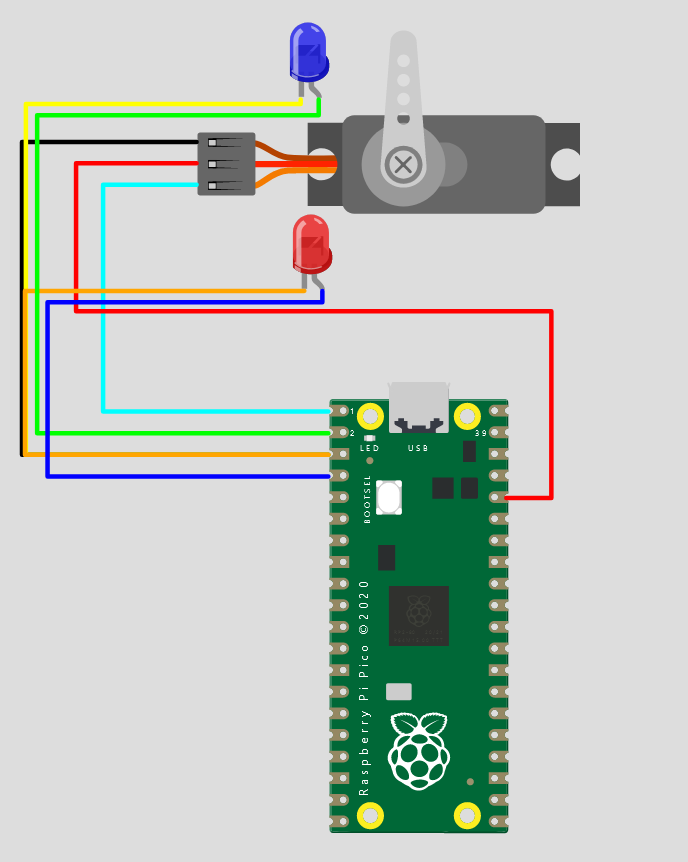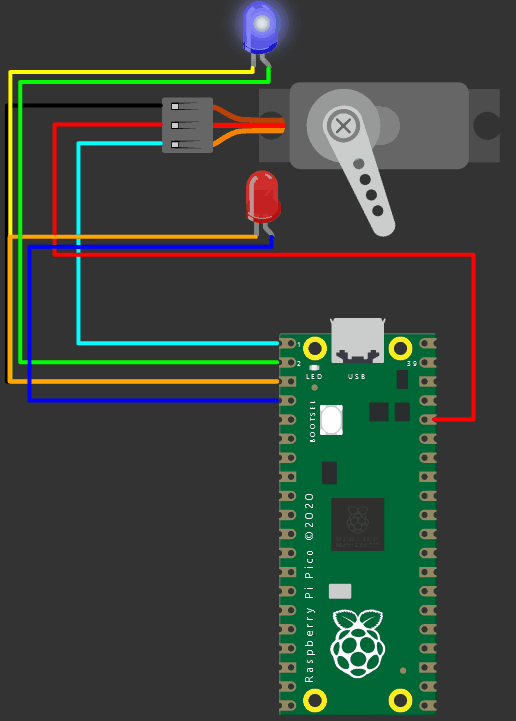Virtual Pi Pico simulator from Wokwi presents you a basic Servo motor drive example along with two LEDs

Raspberry Pi Pico simulator from Wokwi is used in this project. You will learn how to drive the servo motor and also drive two LEDs connected to the Pi Pico in Arduino style programming.

## Pi Pico simulator connection diagram

You will need following items

• Pi Pico (Pi Pico emulator will do)
• Servo motor
• LEDs x 2
• Connecting wiresRaspberry Pi Pico simulator projects - Servo motor control

```        ```/* Sweep
by BARRAGAN <http://barraganstudio.com>
This example code is in the public domain.

modified 8 Nov 2013
by Scott Fitzgerald
http://www.arduino.cc/en/Tutorial/Sweep
*/

#include <Servo.h>

Servo myservo;  // create servo object to control a servo
// twelve servo objects can be created on most boards

int pos = 0;    // variable to store the servo position

void setup() {
myservo.attach(0);  // attaches the servo on pin 9 to the servo object
pinMode(1, OUTPUT);
pinMode(3, OUTPUT);

}

void loop() {
for (pos = 0; pos <= 180; pos += 1) { // goes from 0 degrees to 180 degrees
// in steps of 1 degree
myservo.write(pos);              // tell servo to go to position in variable 'pos'
delay(15);                       // waits 15ms for the servo to reach the position
}
digitalWrite(1, HIGH);   // turn the LED on (HIGH is the voltage level)
delay(15);                       // waits 15ms for the servo to reach the position

digitalWrite(2, LOW);   // turn the LED on (HIGH is the voltage level)

for (pos = 180; pos >= 0; pos -= 1) { // goes from 180 degrees to 0 degrees
myservo.write(pos);              // tell servo to go to position in variable 'pos'
delay(15);                       // waits 15ms for the servo to reach the position
}
digitalWrite(2, HIGH);   // turn the LED on (HIGH is the voltage level)
delay(15);                       // waits 15ms for the servo to reach the position

digitalWrite(1, LOW);   // turn the LED on (HIGH is the voltage level)

}```
```

## Pi Pico simulation - Here is how the project looksWokwi Pi Pico simulator - Servo motor and LED drive

## Live link to the Pi Pico project

https://wokwi.com/arduino/projects/303904623239889474?dark=0